Updating search results...

# 30 Results

View
Selected filters:
• The Mathematics Common Core Toolbox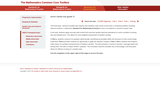Rating
0.0 stars
Overview:

This three-part, machine-scorable task requires that students make sense of and solve a contextual problem involving rational numbers. In the task, students apply securely held content from previous grades (decimal operations) to solve a problem involving rate and elapsed time.

Subject:
Mathematics
Material Type:
Activity/Lab
Interactive
Provider:
The Mathematics Common Core Toolbox
Author:
The Charles A. Dana Center at the University of Texas at Austin and Agile Mind, Inc.
02/26/2019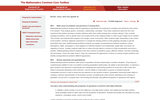Rating
0.0 stars
Overview:

This single-part task illustrates what problem solving means in the CCSSM. Although there are many entry points into this problem, students must analyze constraints, relationships, and goals to find all possible solutions. They formulate a solution pathway rather than jump into a solution attempt.

Subject:
Mathematics
Material Type:
Activity/Lab
Interactive
Provider:
The Mathematics Common Core Toolbox
Author:
The Charles A. Dana Center at the University of Texas at Austin and Agile Mind, Inc.
02/26/2019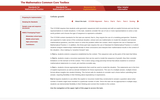Rating
2.0 stars
Overview:

Students consider the use of one or more representations to solve a real-world problem and choose the type of sequence to represent a situation.

Subject:
Math 1
Material Type:
Activity/Lab
Interactive
Provider:
The Mathematics Common Core Toolbox
Author:
The Charles A. Dana Center at the University of Texas at Austin and Agile Mind, Inc.
02/26/2019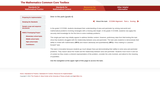Rating
3.0 stars
Overview:

This single-part task may initially appear to address familiar content. However, preliminary data from field testing this task show that students struggled with the relationship between area and perimeter.

Subject:
Mathematics
Material Type:
Activity/Lab
Interactive
Provider:
The Mathematics Common Core Toolbox
Author:
The Charles A. Dana Center at the University of Texas at Austin and Agile Mind, Inc.
02/26/2019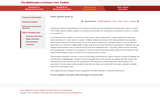Rating
0.0 stars
Overview:

Students can solve the problem using a variety of approaches. Logical mathematical reasoning, rather than reliance on rules, leads to a solution. In Part a, students demonstrate their understanding first by analyzing a visual model to determine a fractional area; they are then asked to determine an equivalent fraction in order to represent that number on a number line. Parts b and c require students to think about fractional representations in different ways and recognize representations that are unlike those they may have traditionally seen. In so doing, students reason abstractly and computationally.

Subject:
Mathematics
Material Type:
Activity/Lab
Interactive
Provider:
The Mathematics Common Core Toolbox
Author:
The Charles A. Dana Center at the University of Texas at Austin and Agile Mind, Inc.
02/26/2019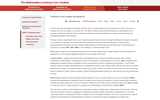Rating
0.0 stars
Overview:

This five-part task illustrates the important CCSSM shift to consider fractions not only as parts of a whole, but also as numbers that can be located on a number line. This latter meaning helps students develop an understanding of the structure of fractions within the number system and builds an important foundation for using number properties and developing operations with fractions.

Subject:
Mathematics
Material Type:
Activity/Lab
Interactive
Provider:
The Mathematics Common Core Toolbox
Author:
The Charles A. Dana Center at the University of Texas at Austin and Agile Mind, Inc.
02/26/2019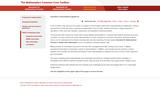Rating
0.0 stars
Overview:

This three-part task involves the critical skill of using multiple representations. It requires that students represent and solve a problem, and then reason from those representations to make comparisons and draw conclusions.

Subject:
Mathematics
Material Type:
Activity/Lab
Interactive
Provider:
The Mathematics Common Core Toolbox
Author:
The Charles A. Dana Center at the University of Texas at Austin and Agile Mind, Inc.
02/26/2019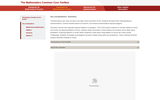Rating
0.0 stars
Overview:

Students explore dilations of lines by selecting points along the line and thinking about point-by-point dilations. Students connect to the definition of a dilation using ratios. The dynamic nature of the animation allows students to explore a variety of situations, including the location of the center of dilation with respect to the line and the scale factor used. Students discover that, if the center of dilation is not on a line, the dilation of that line is a line parallel to the original line.

Subject:
Math 2
Material Type:
Activity/Lab
Interactive
Provider:
The Mathematics Common Core Toolbox
Author:
The Charles A. Dana Center at the University of Texas at Austin and Agile Mind, Inc.
02/26/2019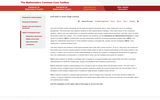Rating
4.5 stars
Overview:

They make sense of the contextual situation, extract and use mathematics to model the situation and answer mathematical questions, and then return to solve the problem within the context.

Subject:
Math 1
Math 3
Material Type:
Activity/Lab
Interactive
Provider:
The Mathematics Common Core Toolbox
Author:
The Charles A. Dana Center at the University of Texas at Austin and Agile Mind, Inc.
02/26/2019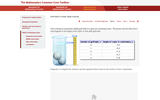Rating
3.5 stars
Overview:

Part A asks students to identify an average rate of change for an approximately linear relationship and write a symbolic representation to model the relationship, using a nontraditional and machine-scored format.

Subject:
Math 1
Math 3
Material Type:
Activity/Lab
Assessment
Provider:
The Mathematics Common Core Toolbox
Author:
The Charles A. Dana Center at the University of Texas at Austin and Agile Mind, Inc.
02/26/2019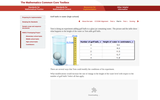Rating
4.0 stars
Overview:

Part B addresses student understanding of the concept of rate of change within the provided context. This item uses a machine-scored, multiple-choice format that requires students to select all correct answers from the set provided. This is designed to elicit deeper analysis than a traditional single-answer, multiple-choice format.

Subject:
Math 1
Math 3
Material Type:
Activity/Lab
Assessment
Provider:
The Mathematics Common Core Toolbox
Author:
The Charles A. Dana Center at the University of Texas at Austin and Agile Mind, Inc.
02/26/2019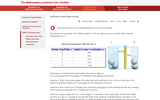Rating
4.5 stars
Overview:

Part C requires students to interpret both parameters, rate-of-change and y-intercept, of the linear functions within the context. It requires significant reasoning about the mathematical ideas within the context and precise communication about the mathematical models.

Subject:
Math 1
Math 3
Material Type:
Activity/Lab
Assessment
Provider:
The Mathematics Common Core Toolbox
Author:
The Charles A. Dana Center at the University of Texas at Austin and Agile Mind, Inc.
02/26/2019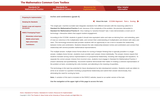Rating
0.0 stars
Overview:

This technology-enhanced task provides students with an opportunity to use a tool to visualize the relationship between inches and centimeters. Students interpret the ratio relationship between inches and centimeters and connect that relationship with several possible mathematical representations.

Subject:
Mathematics
Material Type:
Activity/Lab
Interactive
Provider:
The Mathematics Common Core Toolbox
Author:
The Charles A. Dana Center at the University of Texas at Austin and Agile Mind, Inc.
02/26/2019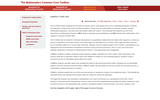Rating
2.0 stars
Overview:

Students consider a financial context requiring multiple calculations for each month, calculate values and complete the table for the second and third months, and extend their use of the recursive process or sequence of steps to find additional values.

Subject:
Math 1
Math 2
Math 3
Material Type:
Activity/Lab
Interactive
Provider:
The Mathematics Common Core Toolbox
Author:
The Charles A. Dana Center at the University of Texas at Austin and Agile Mind, Inc.
02/26/2019Rating
2.0 stars
Overview:

Students explore the technique of completing the square using algebra tiles. At the same time, they build understanding about important characteristics of quadratic functions by connecting the expression that results from completing the square to the vertex of the graph of a quadratic function.

Subject:
Math 1
Math 2
Material Type:
Activity/Lab
Interactive
Provider:
The Mathematics Common Core Toolbox
Author:
The Charles A. Dana Center at the University of Texas at Austin and Agile Mind, Inc.
02/26/2019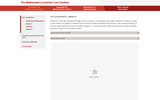Rating
0.0 stars
Overview:

Students consider the relationship between the volume of air in a person's lungs over time as they breathe. Students look at a graphical model and describe key features of the graph. Students notice that the shape of the function is different from the linear, quadratic, and exponential functions they have studied previously. This motivates the need for cubic functions.

Subject:
Math 1
Math 2
Math 3
Material Type:
Activity/Lab
Interactive
Provider:
The Mathematics Common Core Toolbox
Author:
The Charles A. Dana Center at the University of Texas at Austin and Agile Mind, Inc.
02/26/2019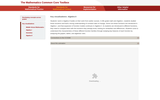Rating
0.0 stars
Overview:

Ideally, students would investigate many different situations that result in a cubic function, such as in the Algebra II example shown on this site in the Standards for Mathematical Practice area. This motivates the need to understand the behavior of the graph of a cubic function.

Subject:
Math 1
Math 2
Math 3
Material Type:
Activity/Lab
Interactive
Provider:
The Mathematics Common Core Toolbox
Author:
The Charles A. Dana Center at the University of Texas at Austin and Agile Mind, Inc.
02/26/2019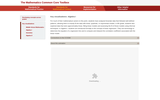Rating
3.0 stars
Overview:

Students are introduced to the concept of the residual. They use a regression equation to compute the values of the residuals for a set of data and see how residuals can be understood by drawing error lines connecting each point in the scatterplot of the data to the residual line. Finally, they plot the residuals and see how their distribution relative to the x-axis can be used to assess the quality of the fit of the linear model.

Subject:
Math 1
Material Type:
Activity/Lab
Interactive
Provider:
The Mathematics Common Core Toolbox
Author:
The Charles A. Dana Center at the University of Texas at Austin and Agile Mind, Inc.
02/26/2019Rating
0.0 stars
Overview:

Students use transformations to show two triangles are similar. Students can use Patty Paper or MIRAs to work in small groups to find a sequence of transformations that will map ABD onto DEF. The key is for students to recognize the need for a reflection and dilation. Once students have found a sequence of transformations, they should develop a viable argument to share with other small groups. As student groups share their arguments, they should be encouraged to use precise language about the transformations and similarity.

Subject:
Math 2
Material Type:
Activity/Lab
Interactive
Provider:
The Mathematics Common Core Toolbox
Author:
The Charles A. Dana Center at the University of Texas at Austin and Agile Mind, Inc.
02/26/2019Rating
0.0 stars
Overview:

This animation provides an example of how an engaging context can be used to engage students in finding common factors and using the greatest common factor prior to formalizing the vocabulary or a particular process. Students are challenged to assemble prize bags, each of which have the same contents.

Subject:
Mathematics
Material Type:
Activity/Lab
Interactive
Provider:
The Mathematics Common Core Toolbox
Author:
The Charles A. Dana Center at the University of Texas at Austin and Agile Mind, Inc.